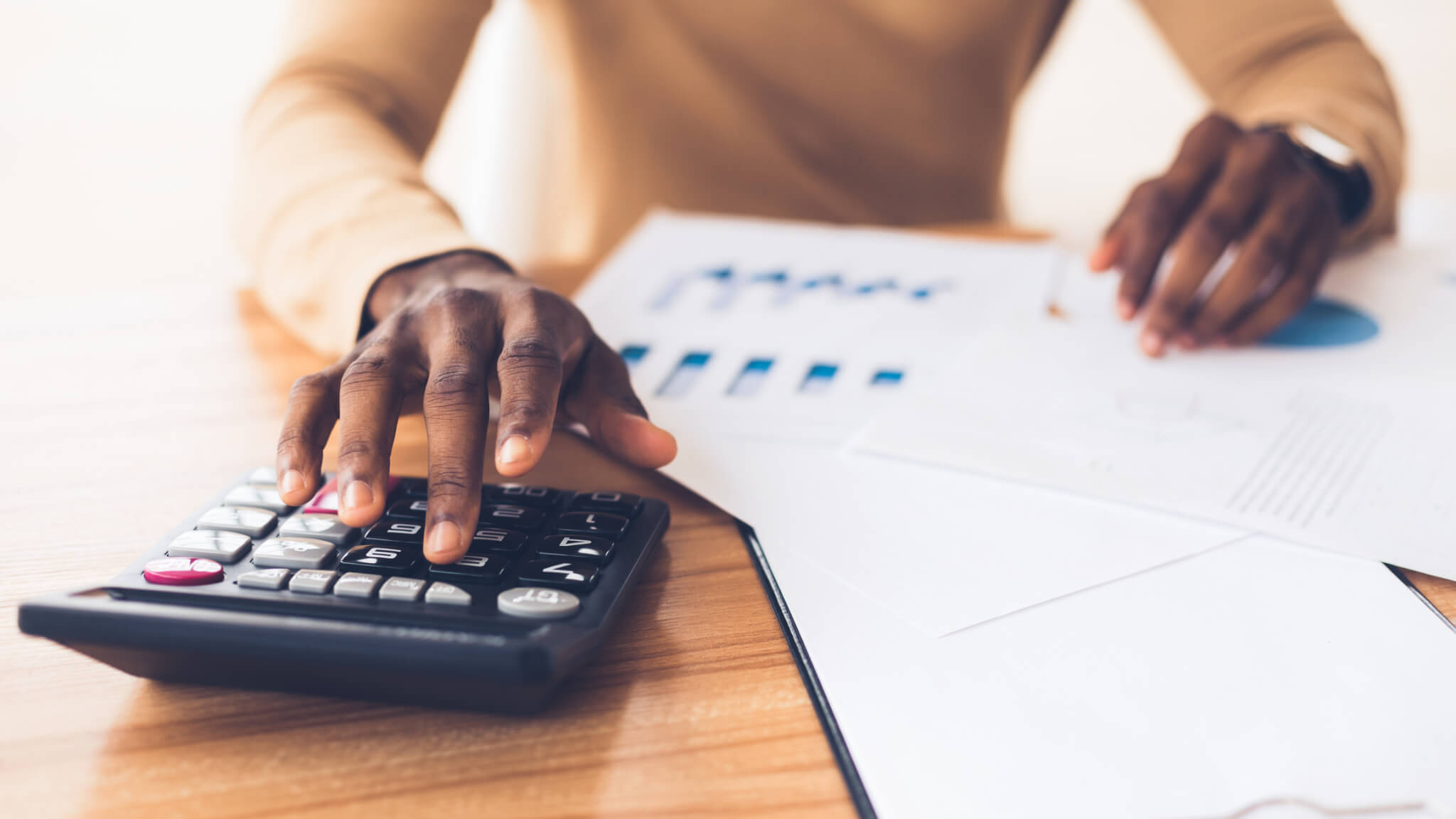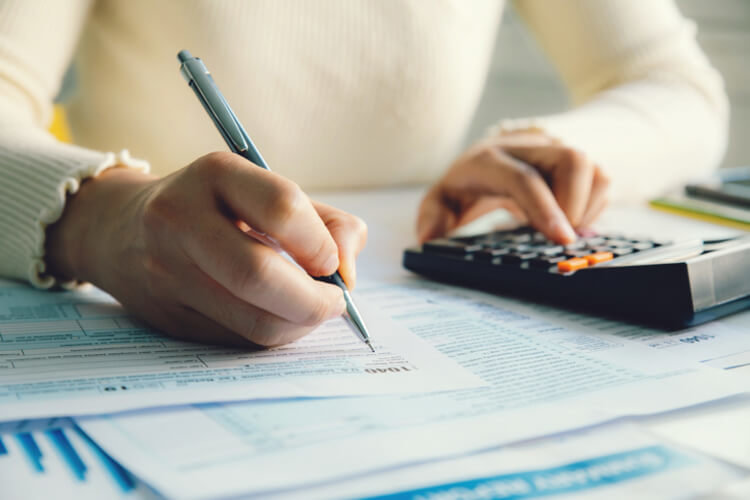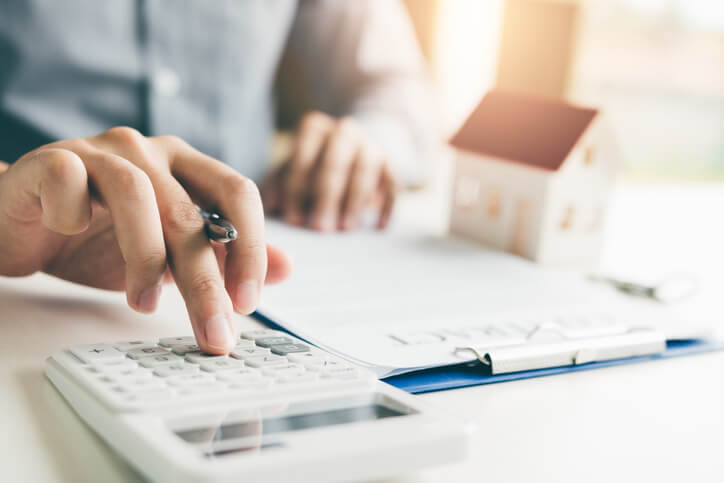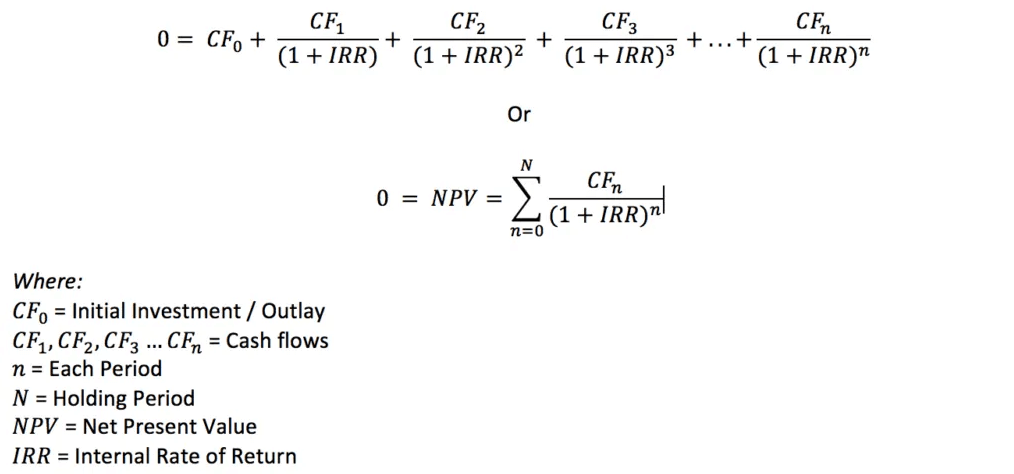# How to Calculate ROI on a Rental Property to Uncover Good Dealsby Jeff Rohde, posted in Investment Strategy

Three main reasons people invest in real estate are for the recurring income, potential appreciation in property value over the long term, and the tax benefits that rental property owners receive.

ROI is one of the key metrics that real estate investors use to choose the best rental property investments that will generate income and help to build wealth year after year.

In this article, we’ll take a look at how ROI works, along with several different ways to calculate ROI on a rental property.

## What is ROI?

ROI (return on investment) measures the profit or gain made on an investment compared to the original cost of the investment, and is expressed as a percentage.

Hard assets such as cash, gold, and real estate all generate different returns for an investor. Here’s the ROI you would earn on each of these three assets, assuming you invested \$100,000 one year ago:

• Cash (in a CD) = 0.17% or \$170
• Gold (physical) = 8.73% or \$8,730
• Real estate (single-family, appreciation only) = 14.1% or \$14,100

(According to Bankrate, Kitco, and Zillow, as of May/June 2021)

The ROI from a CD and physical gold remains the same wherever you are.

However, the return on investment from real estate varies based on the local market conditions, type of property (such as single-family, small multifamily, short-term rental, or commercial real estate), and the before-tax cash flow from the rental income.

For example, values for a single-family home in San Francisco increased by just 1.2% last year, while single-family home values in Austin have grown by 32% over the past 12 months (Zillow through May 2021).## Why ROI Matters for Real Estate Investors

Investing in real estate is one of the best ways that literally anyone can build wealth over the long term.

Property prices historically increase in value faster than the rate of inflation, generate cash flow from rent payments, and have tax benefits such as operating expense and depreciation deductions that other investments simply do not offer all at the same time.

As we’ve seen from the above examples, ROI measures how efficient an investment is in generating returns for an investor, and how potentially lucrative the investment will be over the long term. The more return an investment generates each year, the faster your wealth will grow year after year.

Inflation has a significant effect on how much money you make or lose.

According to the U.S. Bureau of Labor Statistics, the current rate of inflation as measured by the consumer price index (CPI) is 4.2% (April 2021). That means if you hold cash in a CD, your investment is actually losing nearly 4% of its value each year, assuming inflation doesn’t keep going up.

On the other hand, investing in real estate generates a return of about 10% after accounting for inflation, and even more when you factor in the net cash flow from rental property income.

But not all real estate investments generate the same ROI. In the next section, we’ll look at a few different ways to calculate the rate of return on an investment property.## How to Calculate ROI on Rental Property

ROI on a real estate rental property is calculated using the following formula:

• ROI = (Gain on investment – Cost of investment) / Cost of investment

You can invest in real estate using all cash, or by financing the property. Let’s look at the ROI for a cash purchase and a financed purchase, using our \$100,000 in capital. We’ll assume the property is sold after five years for \$135,000:

ROI on a cash purchase

• Purchase price = \$100,000
• Sale price = \$135,000
• Gain on sale = \$35,000
• Mortgage expense = \$0
• Before-tax cash flow = \$6,000 from rental income

Note: we’re not including property tax estimates here as they can vary widely from state to state.

Gain on investment = \$6,000 before tax cash flow x 5 years = \$30,000 + \$35,000 gain on sale = \$65,000 + \$100,000 return of initial investment

• ROI = (\$165,000 – \$100,000) / \$100,000 = 10.53% annualized ROI with total ROI of 65%

ROI on a financed purchase

• Purchase price = \$100,000
• Down payment = \$25,000
• Sale price = \$135,000
• Gain on sale = \$35,000
• Mortgage expense = \$3,804 (principle and interest)
• Before tax cash flow = \$6,000 – \$3,804 = \$2,196

Gain on investment = \$2,196 before tax cash flow x 5 years = \$10,980 + \$35,000 gain on sale = \$45,980

• ROI = (\$45,980 – \$25,000) / \$25,000 = 12.96% annualized ROI with a total ROI of 83.92%

By financing the purchase of an investment property, an investor in this example generates 2.43% more in return on investment each year compared to paying all cash for the property.

ROI on multiple financed purchases

At the beginning of this section, we said that the investor has \$100,000 in capital to invest. By using leverage, four rental properties could be purchased by using a 25% down payment (\$25,000) for each home compared to paying all cash for one house.

Assuming the five-year return on each property was the same, the annualized and total ROIs would still be 12.96% and 83.92% respectively, but the actual total gain on investment from all four properties would be \$183,920:

• \$45,980 gain on investment per property x 4 properties = \$183,920 total gain on investment

By investing in four different properties, an investor can diversify a portfolio geographically by purchasing rental houses in different parts of the country.

ROI on a one-year holding period

The above examples calculated the ROI over a multi-year holding period and the property being sold. You can also use a modified version of the ROI formula to calculate the return on investment for one year when you still own the property:

• ROI = Before-tax cash flow / Total investment

For the all-cash purchase, the one-year ROI is:

• \$6,000 Before-tax cash flow / \$100,000 Total investment = 6.0%

For the financed purchase, the one-year ROI is:

• \$2,196 Before-tax cash flow / \$25,000 Total investment = 8.8%## Other Ways to Calculate Rental Property Returns

In addition to the ROI formula, there are four other ways a real estate investor can calculate rental property returns:

Net Operating Income

Net operating income (NOI) is the cash flow a rental property generates after operating expenses but before the mortgage expense is factored in. When our investor purchased the property for cash, the before-tax cash flow of \$6,000 is the NOI, because there was no mortgage payment.

Cap Rate

Cap rate (capitalization rate) is a calculation used to compare the current or expected returns from similar properties in the same market. The cap rate formula does not factor in the mortgage payment, because different investors purchase and finance rental property differently:

• Cap rate = NOI / Property value
• \$6,000 NOI / \$100,000 Property value = 6%

Real estate investors can also use the cap rate formula to calculate what a property’s value should be based on the market cap rate and NOI, and what the NOI should be based on the market cap rate and property value.

To calculate what the property value should be, rearrange the cap rate formula like this:

• Property value = NOI / Cap rate
• \$6,000 NOI / 6% Cap rate = \$100,000 Property value

To calculate what the NOI should be, rearrange the cap rate formula like this:

• NOI = Property value x Cap rate
• \$100,000 Property value x 6% Cap rate = \$6,000 NOI

Cash-on-Cash Return

Cash-on-cash return compares the annual before-tax cash flow to the total cash invested, and uses the same calculations that the ROI formula for a one-year holding period does:

• Cash-on-cash return = Before-tax cash flow / Total cash invested
• \$2,196 Before-tax cash flow / \$25,000 Total cash invested = 8.8%

Internal Rate of Return

Internal rate of return (IRR) measures the value of the income a property generates during the holding period, taking into account the time value of money. The IRR formula looks like this:(Source: Corporate Finance Institute)

Using the internal rate of return formula, we can calculate the IRR for the property purchased for cash and using financing:

• Cash purchase: \$100,000 purchase price, \$135,000 sale price, \$6,000 before tax annual cash flow = 11.56% IRR
• Financed purchase: \$25,000 down payment, \$135,000 sale price, \$2,196 before tax annual cash flow = 14.75% IRR

While the IRR calculation is a good formula to calculate the potential return from a rental property, it’s also one of the most complicated.

To calculate IRR, you can use an online IRR Calculator or this simple spreadsheet by Roofstock. You can also use that spreadsheet to view projected key return on investment (ROI) metrics, including cash flow, cash-on-cash return, net operating income, and cap rate.

### Final Thoughts on Calculating ROI

ROI, or return on investment, measures how efficient an investment is in generating returns over a period of time. Investing in real estate is something that literally everyone can do to build wealth over the long term.

By calculating the ROI of a potential rental property purchase, real estate investors can choose the best properties that will generate the highest potential returns to increase wealth year after year.